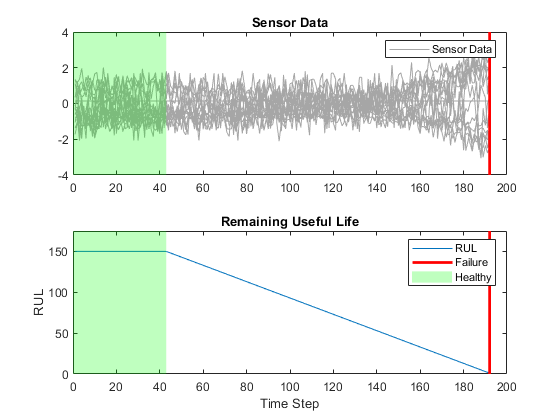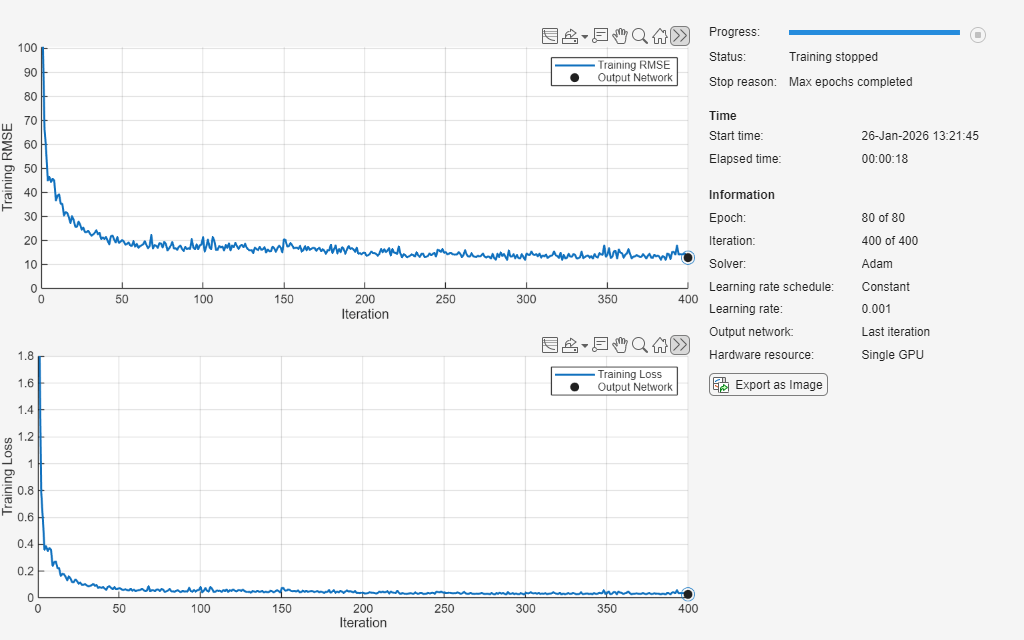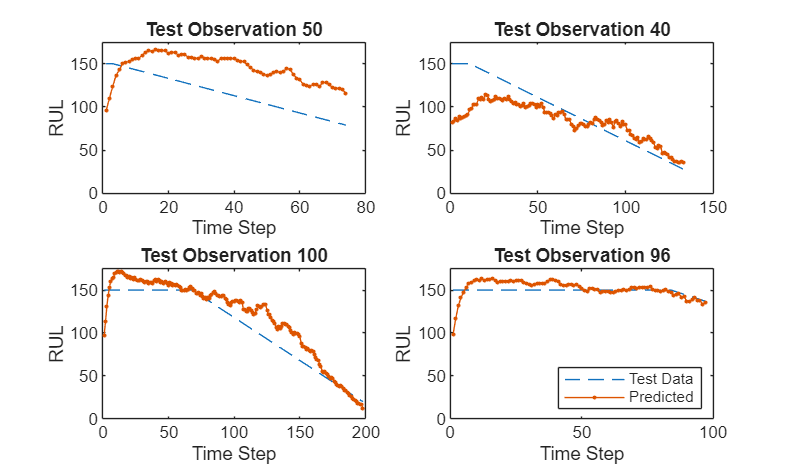# Sequence-to-Sequence Regression Using Deep Learning

This example shows how to predict the remaining useful life (RUL) of engines by using deep learning.

To train a deep neural network to predict numeric values from time series or sequence data, you can use a long short-term memory (LSTM) network.

This example uses the Turbofan Engine Degradation Simulation Data Set as described in . The example trains an LSTM network to predict the remaining useful life of an engine (predictive maintenance), measured in cycles, given time series data representing various sensors in the engine. The training data contains simulated time series data for 100 engines. Each sequence varies in length and corresponds to a full run to failure (RTF) instance. The test data contains 100 partial sequences and corresponding values of the remaining useful life at the end of each sequence.

The data set contains 100 training observations and 100 test observations.

Each time series of the Turbofan Engine Degradation Simulation data set represents a different engine. Each engine starts with unknown degrees of initial wear and manufacturing variation. The engine is operating normally at the start of each time series, and develops a fault at some point during the series. In the training set, the fault grows in magnitude until system failure.

The data contains a ZIP-compressed text files with 26 columns of numbers, separated by spaces. Each row is a snapshot of data taken during a single operational cycle, and each column is a different variable. The columns correspond to the following:

• Column 1 – Unit number

• Column 2 – Time in cycles

• Columns 3–5 – Operational settings

• Columns 6–26 – Sensor measurements 1–21

Create a directory to store the Turbofan Engine Degradation Simulation data set.

```dataFolder = fullfile(tempdir,"turbofan"); if ~exist(dataFolder,'dir') mkdir(dataFolder); end```

Unzip the data from the file `CMAPSSData.zip`.

```filename = "CMAPSSData.zip"; unzip(filename,dataFolder)```

### Prepare Training Data

Load the data using the function `processTurboFanDataTrain` attached to this example. The function `processTurboFanDataTrain` extracts the data from `filenamePredictors` and returns the cell arrays `XTrain` and `YTrain`, which contain the training predictor and response sequences.

```filenamePredictors = fullfile(dataFolder,"train_FD001.txt"); [XTrain,YTrain] = processTurboFanDataTrain(filenamePredictors);```

Remove Features with Constant Values

Features that remain constant for all time steps can negatively impact the training. Find the rows of data that have the same minimum and maximum values, and remove the rows.

```m = min([XTrain{:}],[],2); M = max([XTrain{:}],[],2); idxConstant = M == m; for i = 1:numel(XTrain) XTrain{i}(idxConstant,:) = []; end```

View the number of remaining features in the sequences.

`numFeatures = size(XTrain{1},1)`
```numFeatures = 17 ```

Normalize Training Predictors

Normalize the training predictors to have zero mean and unit variance. To calculate the mean and standard deviation over all observations, concatenate the sequence data horizontally.

```mu = mean([XTrain{:}],2); sig = std([XTrain{:}],0,2); for i = 1:numel(XTrain) XTrain{i} = (XTrain{i} - mu) ./ sig; end```

Clip Responses

To learn more from the sequence data when the engines are close to failing, clip the responses at the threshold 150. This makes the network treat instances with higher RUL values as equal.

```thr = 150; for i = 1:numel(YTrain) YTrain{i}(YTrain{i} > thr) = thr; end```

This figure shows the first observation and the corresponding clipped response.To minimize the amount of padding added to the mini-batches, sort the training data by sequence length. Then, choose a mini-batch size which divides the training data evenly and reduces the amount of padding in the mini-batches.

Sort the training data by sequence length.

```for i=1:numel(XTrain) sequence = XTrain{i}; sequenceLengths(i) = size(sequence,2); end [sequenceLengths,idx] = sort(sequenceLengths,'descend'); XTrain = XTrain(idx); YTrain = YTrain(idx);```

View the sorted sequence lengths in a bar chart.

```figure bar(sequenceLengths) xlabel("Sequence") ylabel("Length") title("Sorted Data")```Choose a mini-batch size which divides the training data evenly and reduces the amount of padding in the mini-batches. Specify a mini-batch size of 20. This figure illustrates the padding added to the unsorted and sorted sequences.

`miniBatchSize = 20;`### Define Network Architecture

Define the network architecture. Create an LSTM network that consists of an LSTM layer with 200 hidden units, followed by a fully connected layer of size 50 and a dropout layer with dropout probability 0.5.

```numResponses = size(YTrain{1},1); numHiddenUnits = 200; layers = [ ... sequenceInputLayer(numFeatures) lstmLayer(numHiddenUnits,'OutputMode','sequence') fullyConnectedLayer(50) dropoutLayer(0.5) fullyConnectedLayer(numResponses) regressionLayer];```

Specify the training options. Train for 60 epochs with mini-batches of size 20 using the solver `'adam'`. Specify the learning rate 0.01. To prevent the gradients from exploding, set the gradient threshold to 1. To keep the sequences sorted by length, set `'Shuffle'` to `'never'`.

```maxEpochs = 60; miniBatchSize = 20; options = trainingOptions('adam', ... 'MaxEpochs',maxEpochs, ... 'MiniBatchSize',miniBatchSize, ... 'InitialLearnRate',0.01, ... 'GradientThreshold',1, ... 'Shuffle','never', ... 'Plots','training-progress',... 'Verbose',0);```

### Train the Network

Train the network using `trainNetwork`.

`net = trainNetwork(XTrain,YTrain,layers,options);`

### Test the Network

Prepare the test data using the function `processTurboFanDataTest` attached to this example. The function `processTurboFanDataTest` extracts the data from `filenamePredictors` and `filenameResponses` and returns the cell arrays `XTest` and `YTest`, which contain the test predictor and response sequences, respectively.

```filenamePredictors = fullfile(dataFolder,"test_FD001.txt"); filenameResponses = fullfile(dataFolder,"RUL_FD001.txt"); [XTest,YTest] = processTurboFanDataTest(filenamePredictors,filenameResponses);```

Remove features with constant values using `idxConstant` calculated from the training data. Normalize the test predictors using the same parameters as in the training data. Clip the test responses at the same threshold used for the training data.

```for i = 1:numel(XTest) XTest{i}(idxConstant,:) = []; XTest{i} = (XTest{i} - mu) ./ sig; YTest{i}(YTest{i} > thr) = thr; end```

Make predictions on the test data using `predict`. To prevent the function from adding padding to the data, specify the mini-batch size 1.

`YPred = predict(net,XTest,'MiniBatchSize',1);`

The LSTM network makes predictions on the partial sequence one time step at a time. At each time step, the network predicts using the value at this time step, and the network state calculated from the previous time steps only. The network updates its state between each prediction. The `predict` function returns a sequence of these predictions. The last element of the prediction corresponds to the predicted RUL for the partial sequence.

Alternatively, you can make predictions one time step at a time by using `predictAndUpdateState`. This is useful when you have the values of the time steps arriving in a stream. Usually, it is faster to make predictions on full sequences when compared to making predictions one time step at a time. For an example showing how to forecast future time steps by updating the network between single time step predictions, see Time Series Forecasting Using Deep Learning.

Visualize some of the predictions in a plot.

```idx = randperm(numel(YPred),4); figure for i = 1:numel(idx) subplot(2,2,i) plot(YTest{idx(i)},'--') hold on plot(YPred{idx(i)},'.-') hold off ylim([0 thr + 25]) title("Test Observation " + idx(i)) xlabel("Time Step") ylabel("RUL") end legend(["Test Data" "Predicted"],'Location','southeast')```

For a given partial sequence, the predicted current RUL is the last element of the predicted sequences. Calculate the root-mean-square error (RMSE) of the predictions, and visualize the prediction error in a histogram.

```for i = 1:numel(YTest) YTestLast(i) = YTest{i}(end); YPredLast(i) = YPred{i}(end); end figure rmse = sqrt(mean((YPredLast - YTestLast).^2)) histogram(YPredLast - YTestLast) title("RMSE = " + rmse) ylabel("Frequency") xlabel("Error")```

### References

1. Saxena, Abhinav, Kai Goebel, Don Simon, and Neil Eklund. "Damage propagation modeling for aircraft engine run-to-failure simulation." In Prognostics and Health Management, 2008. PHM 2008. International Conference on, pp. 1-9. IEEE, 2008.

2. Saxena, Abhinav, Kai Goebel. "Turbofan Engine Degradation Simulation Data Set." NASA Ames Prognostics Data Repository https://ti.arc.nasa.gov/tech/dash/groups/pcoe/prognostic-data-repository/, NASA Ames Research Center, Moffett Field, CA